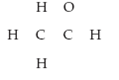×
Get Full Access to Introductory Chemistry - 5 Edition - Chapter 10 - Problem 98p
Get Full Access to Introductory Chemistry - 5 Edition - Chapter 10 - Problem 98p

×

# Write the Lewis structure for CH3COH and determine whether the molecule is polar. DrawISBN: 9780321910295 34

## Solution for problem 98P Chapter 10

Introductory Chemistry | 5th Edition

• Textbook Solutions
• 2901 Step-by-step solutions solved by professors and subject experts
• Get 24/7 help from StudySoup virtual teaching assistantsIntroductory Chemistry | 5th Edition

4 5 1 285 Reviews
13
4
Problem 98P

PROBLEM 98P

Write the Lewis structure for CH3COH and determine whether the molecule is polar. Draw the three-dimensional structure of the molecule. The skeletal structure is:Step-by-Step Solution:

Solution 98P

Step 1:

Here, we have to write the Lewis structure for CH3COH and determine whether the molecule is polar and also Draw the three-dimensional structure of the molecule.

The given skeletal structure is:CH3COH is commonly known as ethanol. It has IUPAC name of Acetaldehyde. Acetaldehyde occurs naturally in coffee, bread, and ripe fruit and is produced by plants. It is also produced by the partial oxidation of ethanol by the liver enzyme alcohol dehydrogenase and may be a contributing factor to hangovers from alcohol consumption.

The lewis structure of Acetaldehyde is given below :Step 2 of 3

Step 3 of 3

##### ISBN: 9780321910295

Introductory Chemistry was written by and is associated to the ISBN: 9780321910295. The answer to “Write the Lewis structure for CH3COH and determine whether the molecule is polar. Draw the three-dimensional structure of the molecule. The skeletal structure is:” is broken down into a number of easy to follow steps, and 24 words. This textbook survival guide was created for the textbook: Introductory Chemistry, edition: 5. The full step-by-step solution to problem: 98P from chapter: 10 was answered by , our top Chemistry solution expert on 05/06/17, 06:45PM. This full solution covers the following key subjects: structure, molecule, coh, draw, lewis. This expansive textbook survival guide covers 19 chapters, and 2046 solutions. Since the solution to 98P from 10 chapter was answered, more than 2086 students have viewed the full step-by-step answer.

Unlock Textbook Solution